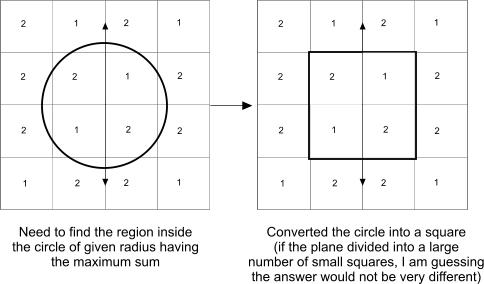2 views

I have a grid (not necessarily a square but I drew it as a square to simplify it) and certain values associated with each of the smaller squares. Given a circle of radius x, I am trying to find the region where the sum of the values is the maximum. The following picture will make it clear:My guess here is that if a plane is divided into a grid using a large number of small squares, approximating the circle to a square will only lead in some over-approximation which is ok to me initially because I have not yet finalized how to address the case of a circle overlapping with a partial square (what would its value be?).

The simplest approach I can think of is brute-force: Start perhaps at the lower left and start moving in a zig-zag path until we hit the top-right and output the region with the maximum sum. I am fine with this approach but for large plane regions, there will be a huge number of squares and at some might, this approach might prove expensive. I am not sure if there is a better way of solving this problem but I would appreciate it if anyone had other thoughts on how to go about solving this.

by (108k points)

I think a brute-force approach might indeed be required if there are trends to the data in the smaller squares. Though you might want to figure out one that wouldn't take the polynomial or exponential time that I believe a naive brute-force approach would require.

However, if there are trends (things like higher values tend to be grouped, or you're more likely to encounter higher values on one side than on another), you could set up an algorithm that would predict regions of your grid that are more likely to contain the higher values.